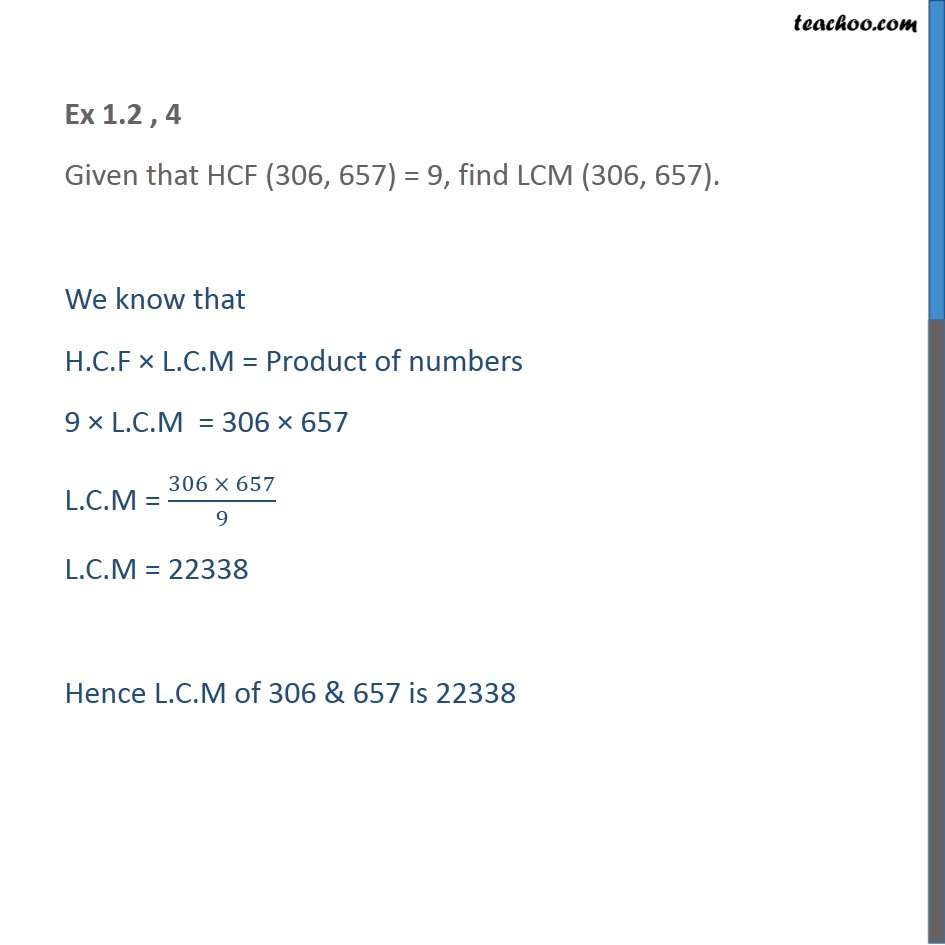Check Important Questions and Chapter Summary -https://you.tube/Real-Numbers-Class-101. Chapter 1 Class 10 Real Numbers
2. Concept wise
3. LCM/HCF

Transcript

Ex 1.2 , 4 Given that HCF (306, 657) = 9, find LCM (306, 657). We know that H.C.F × L.C.M = Product of numbers 9 × L.C.M = 306 × 657 L.C.M = (306 × 657)/9 L.C.M = 22338 Hence L.C.M of 306 & 657 is 22338

LCM/HCF